## New Design Method: Information Outage Minimization

For a fixed rotation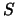and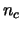fixed MIMO channel matrices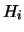,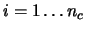, defined by thefading blocks, let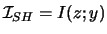denote the average mutual information of the equivalent channel with QAM input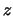and complex output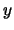as in (1). The expression of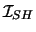is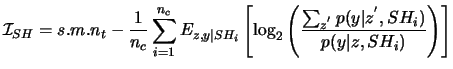(6)

where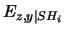is the conditional mathematical expectation overand. The channel likelihood is written in its classical form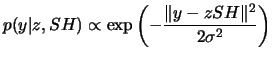(7)

Expression (6) assumes that the precoderdoes space-time spreading within the same fading block. Its main role is to collect transmit diversity. Time diversityis collected by the convolutional code whereas receive diversity is naturally collected by the detector. The information rate transmitted by the space-time BICM is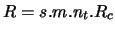bits per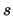time periods. An outage occurs if the instantaneous capacity, i.e.in our case, is less than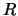. The outage probability associated to the rotationat a given signal-to-noise ratio is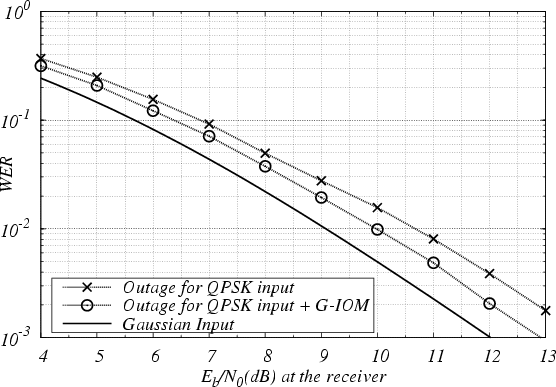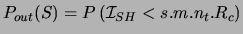(8)

The new design, called IOM (Information Outage Minimization), selects a matrix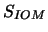within the ensemble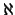of random unitary matrices such that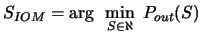(9)

As an example, choosing the best rotation within an ensemblelimited to 2000 matrices yields the matrix written below, for QPSK alphabet with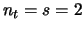and coding rate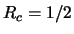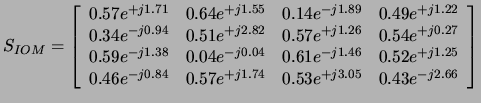A smaller set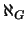of random unitary matrices is obtained by adding tothe first genie constraint, i.e. orthogonal sub-rows in. This second design, called G-IOM, selects a matrix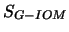satisfying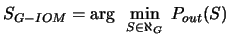(10)

As an example, choosing the best rotation within an ensemblelimited to 2000 matrices yields the matrix written below, for QPSK alphabet withand coding rate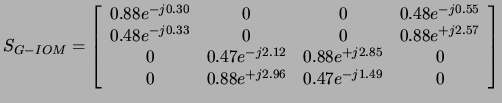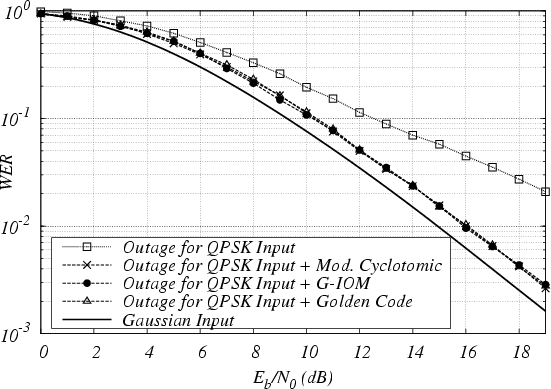In a similar fashion, a DNA-IOM precoder minimizes the information outage and satisfies DNA constraints . The matrix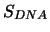given below corresponds to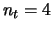and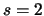. The DNA-IOM precoder is obtained by combiningwith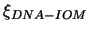. Also, the DNA cyclotomic precoder is constructed by combiningto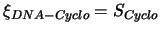given previously in (3).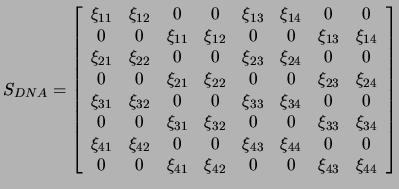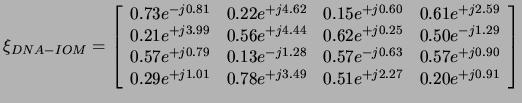Figures 2 and 3 show the outage limit for different type of precoders in terms of Word Error Rate versus signal-to-noise ratio. The outage probability has been also evaluated for other system parameters. Surprisingly, algebraic precoders satisfying the first genie condition perform as good as G-IOM precoding. Cyclotomic rotation and Golden code are superimposed with G-IOM in Figure 2. All outage evaluations have been made by (6) and (8), without gaussian and analytical approximations when the channel input is a gaussian alphabet as in .
Joseph Jean Boutros 2005-05-07